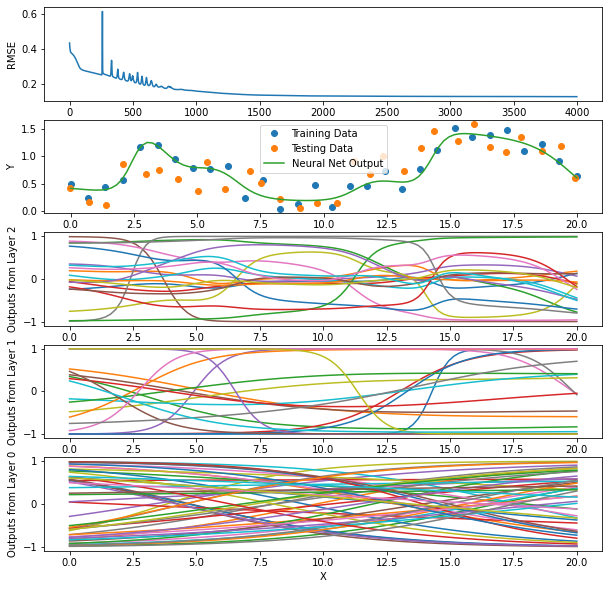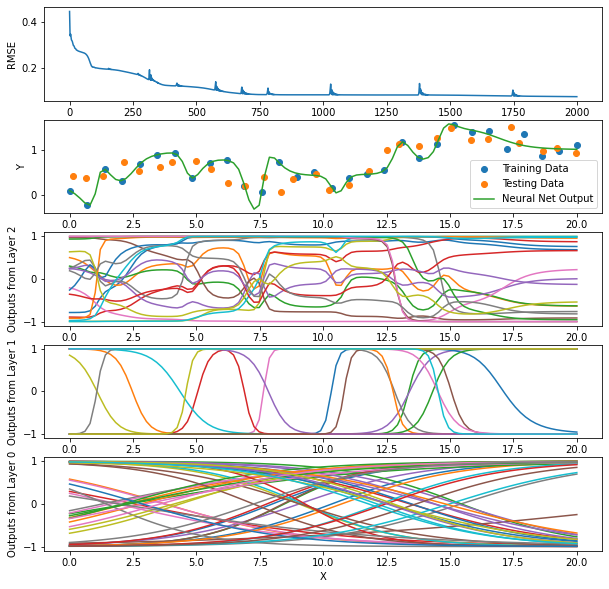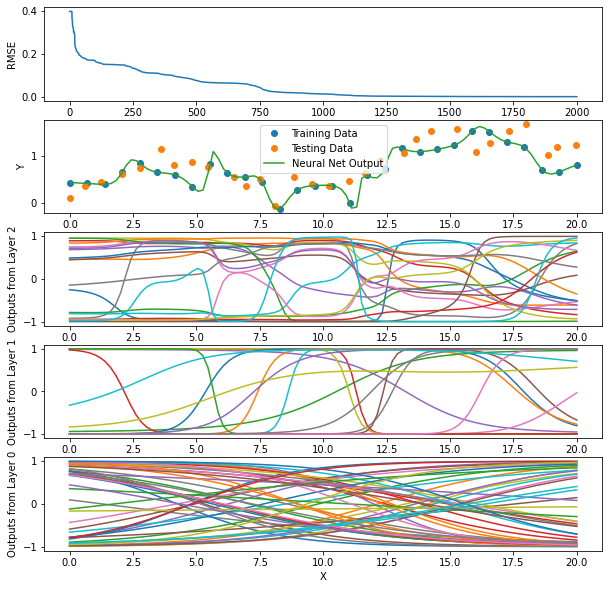# Neural Network Class¶

• A3.3: *Modified A3grader.tar to correctly grade the error_trace value. It now assumes you use the following function for the error_convert_f:

  def error_convert(err):
if T.shape == 1:
return np.sqrt(err) * self.stand_params['Tstds']
else:
# Can't unstandardize err if more than one network output
return np.sqrt(err)
• A3.2: Added A3grader.py and additional requirements involving application of your NeuralNetwork class to a specific data set.

• A3.1: Added some details on specifications of required functions and many examples of running your implementation.

You will define a new class named NeuralNetwork that constructs a neural network with any number of hidden layers. To train the neural network, you will use our optimizers.py code. Use this updated version: optimizers.tar. Your class must implement at least the following functions. Lecture Notes 07 provide examples of some of the code in the section about using Optimizers on which you can base your implementation.

• __init__(self, n_inputs, n_hiddens_list, n_outputs):
• __repr__(self):
• make_weights(self): called from constructor __init__
• initialize_weights(self): called from constructor __init__
• train(self, X, T, n_epochs, learning_rate, method='adam', verbose=True): method can be 'sgd', 'adam', or 'scg'. Must first calculate standarization parameters, stored in stand_params dictionary, and standardize X and T. Import optimizers.py and use these optimizers in this train function. Use the tanh activation function.
• use(self, X, return_hidden_layer_outputs=False): standardizes X then calculates the output of the network by calling forward and unstandardizing the network output. Returns just the output of the last layer. If return_hidden_layer_outputs is True, return two things, the output of the last layer and a list of outputs from each hidden layer.
• get_error_trace(self): just returns the error_trace

Here are some example uses of your NeuralNetwork class. Your implementation should return very close to the same values in each code cell.

In :
import numpy as np
import matplotlib.pyplot as plt

In :
np.random.seed(123)
nnet = NeuralNetwork(1, , 2)  # 2 outputs
nnet

Out:
NeuralNetwork(1, , 2)
In :
nnet.all_weights

Out:
array([ 0.27784939, -0.30244465, -0.38629038,  0.07257004,  0.31037599,
-0.10874389,  0.4807642 ,  0.18482974, -0.0190681 , -0.10788248,
-0.15682198,  0.22904971, -0.06142776, -0.4403221 ])
In :
nnet.Ws

Out:
[array([[ 0.27784939, -0.30244465, -0.38629038],
[ 0.07257004,  0.31037599, -0.10874389]]),
array([[ 0.4807642 ,  0.18482974],
[-0.0190681 , -0.10788248],
[-0.15682198,  0.22904971],
[-0.06142776, -0.4403221 ]])]
In :
nnet.all_gradients

Out:
array([0., 0., 0., 0., 0., 0., 0., 0., 0., 0., 0., 0., 0., 0.])
In :
nnet.Gs

Out:
[array([[0., 0., 0.],
[0., 0., 0.]]),
array([[0., 0.],
[0., 0.],
[0., 0.],
[0., 0.]])]
In :
X = np.linspace(-1, 1, 4).reshape(-1, 1)
X

Out:
array([[-1.        ],
[-0.33333333],
[ 0.33333333],
[ 1.        ]])
In :
T = np.hstack((X ** 2, (X - 2) ** 3))
T

Out:
array([[  1.        , -27.        ],
[  0.11111111, -12.7037037 ],
[  0.11111111,  -4.62962963],
[  1.        ,  -1.        ]])
In :
nnet.train(X, T, 5, 0.01, method='adam')

Adam: Epoch 1 Error=0.46500

Out:
NeuralNetwork(1, , 2)
In :
nnet.stand_params

Out:
{'Xmeans': array([-5.55111512e-17]),
'Xstds': array([0.74535599]),
'Tmeans': array([  0.55555556, -11.33333333]),
'Tstds': array([0.44444444, 9.98799004])}
In :
nnet.Ws

Out:
[array([[ 0.32805609, -0.25283707, -0.33680913],
[ 0.02237977,  0.36049589, -0.15868899]]),
array([[ 0.43097923,  0.13534218],
[-0.06903616, -0.15739471],
[-0.10716161,  0.27902101],
[-0.01180826, -0.44858975]])]
In :
nnet.get_error_trace()

Out:
[0.46500122030518537,
0.4598753839375639,
0.45485689400995843,
0.4498806080959408,
0.4449391314653806]
In :
nnet.use(X)

Out:
array([[  0.76872931, -11.63174677],
[  0.75768845, -10.3937065 ],
[  0.74348724,  -9.01615633],
[  0.72838516,  -7.64787959]])
In :
Y, Zs = nnet.use(X, return_hidden_layer_outputs=True)
Y, Zs  # Zs is list of hidden layer output matrices

Out:
(array([[  0.76872931, -11.63174677],
[  0.75768845, -10.3937065 ],
[  0.74348724,  -9.01615633],
[  0.72838516,  -7.64787959]]),
[array([[ 0.2895092 , -0.62702167, -0.12327529],
[ 0.30774044, -0.39191092, -0.25975089],
[ 0.32574848, -0.09136292, -0.38658353],
[ 0.34352322,  0.22680528, -0.50030489]])])

Now here is a function you may use to train a NeuralNetwork using a particular method and values for the number of epochs and learning rate.

In :
def run(method, n_epochs, learning_rate=0):

n_samples = 30
Xtrain = np.linspace(0., 20.0, n_samples).reshape((n_samples, 1))
Ttrain = 0.2 + 0.05 * (Xtrain) + 0.4 * np.sin(Xtrain / 2) + 0.2 * np.random.normal(size=(n_samples, 1))

Xtest = Xtrain + 0.1 * np.random.normal(size=(n_samples, 1))
Ttest = 0.2 + 0.05 * (Xtest) + 0.4 * np.sin(Xtest / 2) + 0.2 * np.random.normal(size=(n_samples, 1))

n_inputs = Xtrain.shape
n_hiddens_list = [50, 20, 20]
n_outputs = Ttrain.shape

nnet = NeuralNetwork(n_inputs, n_hiddens_list, n_outputs)
nnet.train(Xtrain, Ttrain, n_epochs, learning_rate, method=method, verbose=False)

def rmse(Y, T):
error = T - Y
return np.sqrt(np.mean(error ** 2))

Ytrain = nnet.use(Xtrain)
rmse_train = rmse(Ytrain, Ttrain)
Ytest = nnet.use(Xtest)
rmse_test = rmse(Ytest, Ttest)

print(f'Method: {method}, RMSE: Train {rmse_train:.2f} Test {rmse_test:.2f}')

plt.figure(1, figsize=(10, 10))
plt.clf()

n_plot_rows = nnet.n_layers + 1
ploti = 0

ploti += 1
plt.subplot(n_plot_rows, 1, ploti)
plt.plot(nnet.get_error_trace())
plt.xlabel('Epoch')
plt.ylabel('RMSE')

ploti += 1
plt.subplot(n_plot_rows, 1, ploti)
plt.plot(Xtrain, Ttrain, 'o', label='Training Data')
plt.plot(Xtest, Ttest, 'o', label='Testing Data')
X_for_plot = np.linspace(0, 20, 100).reshape(-1, 1)
Y, Zs = nnet.use(X_for_plot, return_hidden_layer_outputs=True)
plt.plot(X_for_plot, Y, label='Neural Net Output')
plt.legend()
plt.xlabel('X')
plt.ylabel('Y')

for layeri in range(nnet.n_layers - 2, -1, -1):
ploti += 1
plt.subplot(n_plot_rows, 1, ploti)
plt.plot(X_for_plot, Zs[layeri])
plt.xlabel('X')
plt.ylabel(f'Outputs from Layer {layeri}')

return nnet

In :
run('sgd', 4000, 0.1)

Method: sgd, RMSE: Train 0.13 Test 0.29

Out:
NeuralNetwork(1, [50, 20, 20], 1)In :
run('adam', 2000, 0.01)

Method: adam, RMSE: Train 0.07 Test 0.26

Out:
NeuralNetwork(1, [50, 20, 20], 1)In :
run('scg', 2000)

Method: scg, RMSE: Train 0.00 Test 0.31

Out:
NeuralNetwork(1, [50, 20, 20], 1)# QSAR aquatic toxicity Data Set¶

Download the QSAR data set from this UCI ML Repository site. It consists of eight measurements of water quality that may affect a ninth measurement, of aquatic toxicity towards Daphnia Magna.

• read this data into a numpy array,
• randomly shuffle the order of the rows in this data array (np.random.shuffle)
• take the first 500 rows as training data and the remaining rows as testing data,
• assign the first eight columns to Xtrain and Xtest, and the last column to Ttrain and Ttest,
• do some experimentation with different values of n_hiddens_list, n_epochs and learning_rate for the sgd and adam methods, and with different values of n_hiddens_list, n_epochs for scg, which does not use the learning_rate.
• using the parameter values (n_hiddens_list, n_epochs and learning_rate) that you find produce the lowest RMSE on test data for each method, create plots for each method that include the error_trace, the training data targets and predictions by the neural network, and the testing data targets and predictions by the neural network. The differrent methods may use different parameter values.

Describe your results with at least 10 sentences.

Here is an example of the plots we expect to see.Your notebook will be run and graded automatically. Test this grading process by first downloading A3grader.tar and extract A3grader.py from it. Run the code in the following cell to demonstrate an example grading session. The remaining 40 points will be based on other testing and the results you obtain and your discussions.

A different, but similar, grading script will be used to grade your checked-in notebook. It will include additional tests. You should design and perform additional tests on all of your functions to be sure they run correctly before checking in your notebook.

For the grading script to run correctly, you must first name this notebook as 'Lastname-A3.ipynb' with 'Lastname' being your last name, and then save this notebook.

In :
%run -i A3grader.py

======================= Code Execution =======================

Extracting python code from notebook named 'Anderson-A3.ipynb' and storing in notebookcode.py
Removing all statements that are not function or class defs or import statements.
CRITICAL ERROR: Function named 'NeuralNetworks' is not defined
Check the spelling and capitalization of the function name.

## Testing constructor ####################################################################

nnet = NeuralNetwork(2, [5, 4], 3)
W_shapes = [W.shape for W in nnet.Ws]

--- 5/5 points. W_shapes is correct value of [(3, 5), (6, 4), (5, 3)]

## Testing constructor ####################################################################

G_shapes = [G.shape for G in nnet.Gs]

--- 5/5 points. G_shapes is correct value of [(3, 5), (6, 4), (5, 3)]

## Testing constructor ####################################################################

nnet.Ws[2, 0] = 100.0
# Does nnet.Ws[2, 0] == nnet.all_weights

--- 10/10 points. nnet.Ws[2, 0] equals nnet.all_weights

## Testing constructor ####################################################################

G_shapes = [G.shape for G in nnet.Gs]

--- 10/10 points. G_shapes is correct value of [(3, 5), (6, 4), (5, 3)]

## Testing train ####################################################################

Now with two outputs (columns in T)

X = np.arange(20).reshape(-1, 2) + 5
T = np.hstack((X[:, 0:1] * 0.4, (X[:, 1:2] / 10) ** 3))

np.random.seed(123)
nnet = NeuralNetwork(2, [5, 4, 3], 2)
nnet.train(X, T, 1, 0.01, method='sgd')

Check nnet.Gs

Then check  nnet.get_error_trace()

sgd: Epoch 1 Error=1.01668

--- 10/10 points. Correct values in nnet.Gs

--- 10/10 points. Returned correct error_trace [1.0166788679812657]

## Testing train all methods ####################################################################

Now with two outputs (columns in T)

X = np.arange(20).reshape(-1, 2) + 5
T = np.hstack((X[:, 0:1] * 0.4, (X[:, 1:2] / 10) ** 3))

np.random.seed(123)
nnet_sgd = NeuralNetwork(2, [5, 4, 3], 2)
nnet_sgd.train(X, T, 1000, 0.01, method='sgd')

np.random.seed(123)
nnet_adam = NeuralNetwork(2, [5, 4, 3], 2)

np.random.seed(123)
nnet_scg = NeuralNetwork(2, [5, 4, 3], 2)
nnet_scg.train(X, T, 1000, None, method='scg')  # learning_rate is None, not used by scg

def rmse(Y, T):
return np.sqrt(np.mean((T - Y)**2))

rmse_sgd = rmse(nnet_sgd.use(X), T)
rmse_scg = rmse(nnet_scg.use(X), T)

sgd: Epoch 100 Error=0.94423
sgd: Epoch 200 Error=0.65819
sgd: Epoch 300 Error=0.36545
sgd: Epoch 400 Error=0.30044
sgd: Epoch 500 Error=0.27858
sgd: Epoch 600 Error=0.26216
sgd: Epoch 700 Error=0.24840
sgd: Epoch 800 Error=0.23671
sgd: Epoch 900 Error=0.22675
sgd: Epoch 1000 Error=0.21820
SCG: Epoch 100 Error=0.01643
SCG: Epoch 200 Error=0.01003
SCG: Epoch 300 Error=0.00371
SCG: Epoch 400 Error=0.00204
SCG: Epoch 500 Error=0.00161
SCG: Epoch 600 Error=0.00116
SCG: Epoch 700 Error=0.00104
SCG: Epoch 800 Error=0.00099
SCG: Epoch 900 Error=0.00087
SCG: Epoch 1000 Error=0.00083

--- 10/10 points. Correct rmse values.

======================================================================
A3 Execution Grade is 60 / 60
======================================================================

__ / 10 points. Reading data and defining Xtrain, Ttrain, Xtest, Ttest.

__ / 10 points. Experiments with variety of values for n_hiddens_list, n_epochs, and learning_rate
for the three optimization methods.

__ / 10 points. Plots of your best results each of the three methods.

__ / 10 points. Good discussion of results you get, using at least 10 sentences.

======================================================================
A3 Results and Discussion Grade is ___ / 40
======================================================================

======================================================================
A3 FINAL GRADE is  _  / 100
======================================================================


# Check-In¶

Do not include this section in your notebook.

Name your notebook Lastname-A3.ipynb. So, for me it would be Anderson-A3.ipynb. Submit the file using the Assignment 3 link on Canvas.

# Extra Credit¶

Earn one extra credit point by downloading a second, real data set and repeating the above experiments.